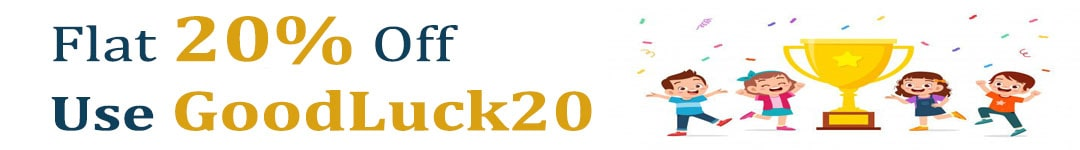International Maths Olympiad Forum By SOF Olympiad Trainer - Page 330

# User ForumSubject :IMO    Class : Class 7

Class : Class 8

## Ans 2:

Class : Class 10
No answer is 2000 sorry

Subject :IMO    Class : Class 7

Class : Class 9
c is correct

## Ans 2:

Class : Class 7
C is the correct answer.

Class : Class 8
c is correct

## Ans 4:

Class : Class 8
Answer is (C)Diameter of bigger circle=28 cmradius=28/2=14 cmArea of semicircle=1/2*22/7*14*14=4312/14=308 sq.cm2 semicircle=circleArea=22/7*7*7=1078/7=154 sq.cmArea left=308-154=154 cm

## Ans 5:

Class : Class 4
option C,154 is correct answer

Class : Class 8

## Ans 7:

Class : Class 8
c is correct308-154=154 you have done this308 154=462hence option c is correct

## Ans 8:

Class : Class 8
Yes , i agree ...Option c is the ans.

Class : Class 8
c is correct

## Ans 10:

Class : Class 5
option third is right

Class : Class 8

Class : Class 9

Class : Class 8
C is correct

Class : Class 8
c is correct

Class : Class 6

## Ans 16:

Class : Class 10
Option C is the answer

Class : Class 10

Class : Class 8
C for corecct

## Ans 19:

Class : Class 8
Here the given answer option D is wrong . The correct answer is option C.

## Ans 20:

Class : Class 9
C is correct

Subject :IMO    Class : Class 8

## Ans 1:

Class : Class 9
√3844=62 Hence the answer is c

Subject :IMO    Class : Class 7

## Ans 1:

Class : Class 7
let the price of object B be x the the price of object A is (6x-100) now ATP, 1(6x-100) + 4*x=1400 =>6x-100 + 4x=1400 =>10x -100=1400 =>10x=1500 =>x=1500/10 =>x=150 now the price of object A=150*6-100 =800(d)

Subject :IMO    Class : Class 10

Class : Class 10
D

## Ans 2:

Class : Class 7
IT IS D BECAUSE 3abc IS equal to A CUBE+B CUBE+C CUBE

## Ans 3:

Class : Class 10
D Because a+b+c=0 And, a3+b3+c3=3abc

## Ans 4:

Class : Class 10
Answer is 3abc cause'. Centroid in coordinate geometry is equal tO X1+X2+X3/2 , Y1+Y2+Y3/2 THEREFORE 0 EQUAL TO X CORDINATE ANT SO A+B+C EQUAL TO ZERO

Subject :IMO    Class : Class 10

#### View All Answers (0)

Subject :IMO    Class : Class 3

Class : Class 6

Class : Class 5

Class : Class 5

## Ans 4:

Class : Class 5
Please correct the question

## Ans 5:

Class : Class 6
Please correct the question. The values to be obtained are not given properly

## Ans 6:

Class : Class 6
Please correct the question , Find the value of star, smiley, pentagon respectively.

Subject :IMO    Class : Class 4

## Ans 1:

Class : Class 7
69

Subject :IMO    Class : Class 6

## Ans 1:

Class : Class 7
If *sun means sum ,then how there be a minus symbol??

## Ans 2:

Class : Class 7
None of them. The answer is 1568568567

Subject :IMO    Class : Class 4

Class : Class 4
A

## Ans 2:

Class : Class 7
there are 10 lines

Class : Class 7
A

Class : Class 7

Class : Class 4
how come A?

## Ans 6:

Class : Class 5
14sleeping lines 42 total lines